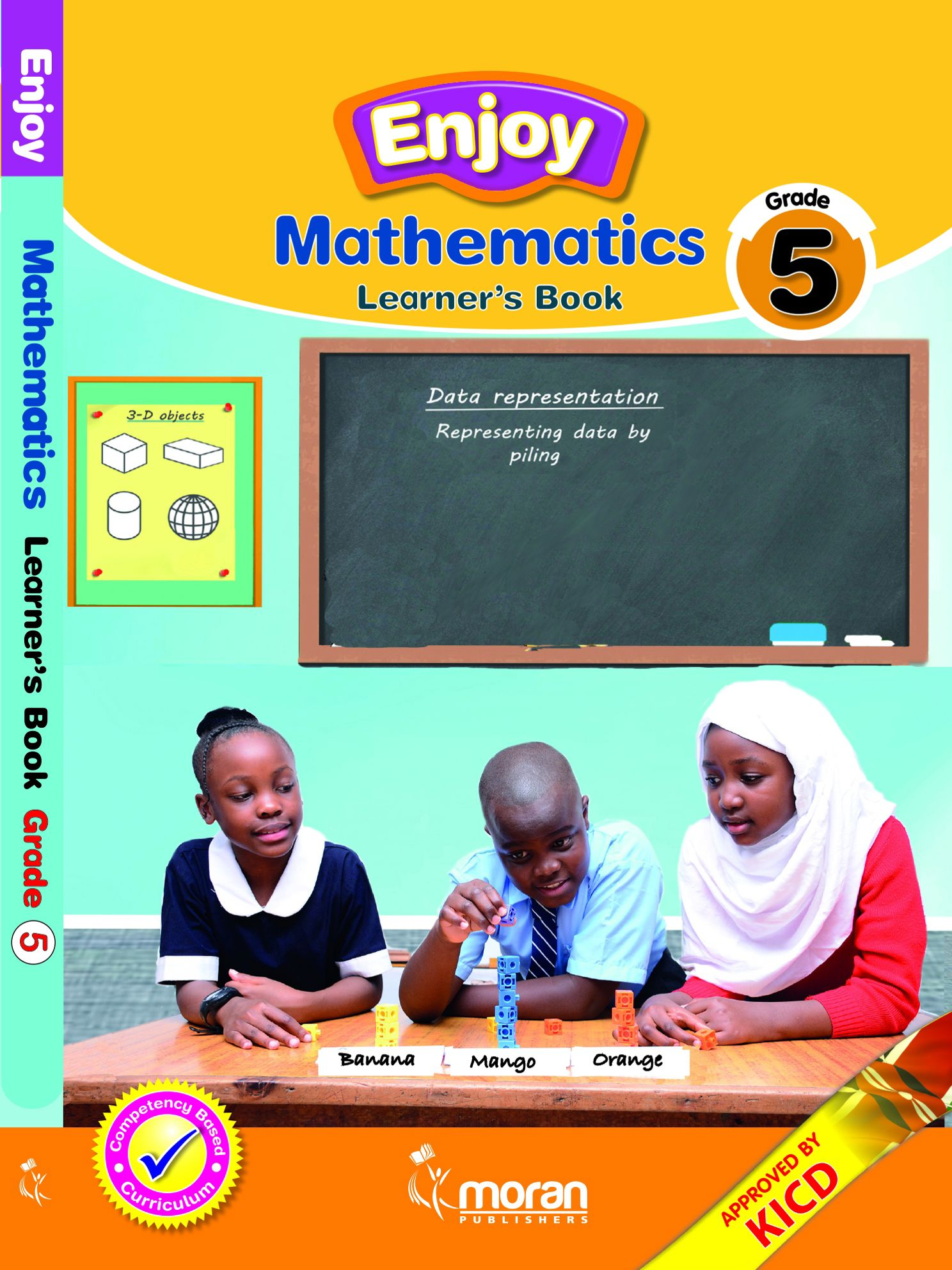Study kit (Mathematics 5th)##### Authors
Paul Njoga, Geoffrey Gichuki, Nickson Oshome, Maina Kiragu
##### Exercises kit’s authors
Paul Njoga, Geoffrey Gichuki, Nickson Oshome, Maina Kiragu
Moran Publishers
##### Included in packages• The study kit contains 108 chapters and teacher’s exercises kit contains 107 exercises.
• ##### Authors
Paul Njoga, Geoffrey Gichuki, Nickson Oshome, Maina Kiragu
• ##### Exercises kit’s authors
Paul Njoga, Geoffrey Gichuki, Nickson Oshome, Maina Kiragu
Mathematics
English
• ##### Publisher
Moran Publishers

### 1. Numbers – Whole numbers

##### Chapter
1.1. Place value
Free chapter!
1.2. Total value
1.3. Writing numbers in symbols
1.4. Reading and writing numbers in words
1.5. Reading and writing numbers in symbols
1.6. Arranging numbers
1.7. Rounding off numbers
1.8. Divisibility by 2 and 5
1.9. Divisibility by 10
1.10. Factors and highest common factor
1.11. Divisors and common divisors
1.12. Greatest common divisor (GCD)
1.13. Multiples and common multiples
1.14. Least common multiple (LCM)

##### Chapter
2.1. Addition of two numbers without regrouping
2.2. Addition of three numbers without regrouping
2.3. Addition of two numbers with double regrouping
2.4. Estimating sums by rounding off numbers to the nearest hundred
2.5. Estimating sums by rounding off numbers to the nearest thousand

### 3. Numbers – Subtraction

##### Chapter
3.1. Subtraction without regrouping
3.2. Subtraction with regrouping
3.3. Estimating difference by rounding off numbers to the nearest hundred
3.4. Estimating difference by rounding off numbers to the nearest thousand
3.5. Combined operations on numbers
3.6. Subtraction patterns

### 4. Numbers – Multiplication

##### Chapter
4.1. Multiplication of 2‑digit numbers
4.2. Multiplication of a 3‑digit number
4.3. Estimating products by rounding off 2‑digit factors to the nearest ten
4.4. Estimating products by rounding off 2‑digit and 3‑digit factors to the nearest ten
4.5. Multiplication patterns (1)
4.6. Multiplication patterns (2)

### 5. Numbers – Division

##### Chapter
5.1. Division of 2‑digit numbers
5.2. Division of a 3‑digit number
5.3. Relationship between multiplication and division
5.4. Estimating quotients
5.5. Combined operations

### 6. Numbers – Fractions

##### Chapter
6.1. Equivalent fractions
6.2. Simplifying fractions
6.3. Comparing fractions
6.4. Ordering fractions
6.5. Adding fractions with the same denomi­nators
6.6. Subtracting fractions with the same denomi­nators
6.8. Subtracting fractions with renaming

### 7. Numbers – Decimals

##### Chapter
7.1. Place value of decimals
7.2. Arranging decimals
7.3. Addition of decimals without regrouping
7.4. Addition of decimals with regrouping
7.5. Subtraction of decimals without regrouping
7.6. Subtraction of decimals with regrouping

### 8. Measurement – Length

##### Chapter
8.1. Kilometre
8.2. Estimating and measuring distance
8.3. Converting kilometres to metres and vice versa
8.6. Subtraction without regrouping
8.7. Subtraction with regrouping
8.8. Multiplication without regrouping
8.9. Multiplication with regrouping
8.10. Division

### 9. Measurement – Area

##### Chapter
9.1. Square centimetre and area of a rectangle
9.2. Area of a square

### 10. Measurement – Volume

##### Chapter
10.1. Cubic centimetre and volume of a cube
10.2. Volume of a cuboid
10.3. Deriving formula for the volume

### 11. Measurement – Capacity

##### Chapter
11.1. Measuring capacity
11.2. Estimating and measuring capacity
11.3. Converting litres to millilitres and vice versa
11.6. Subtraction without regrouping
11.7. Subtraction with regrouping
11.8. Multiplication
11.9. Division

### 12. Measurement – Mass

##### Chapter
12.1. Measuring mass
12.2. Estimating and measuring mass
12.3. Converting kilograms to grams and vice versa
12.6. Subtraction without regrouping
12.7. Subtraction with regrouping
12.8. Multiplication
12.9. Division

### 13. Measurement – Time

##### Chapter
13.1. Second and minute
13.2. Converting minutes to seconds and vice versa
13.4. Subtraction
13.5. Multiplication
13.6. Division

14.1. Budget
14.2. Tax
14.3. Bank
14.4. Saving

### 15. Geometry – Lines

##### Chapter
15.1. Horizontal and vertical lines
15.2. Perpendicular lines
15.3. Parallel lines

### 16. Geometry – Angles

##### Chapter
16.1. Relating a turn to angles
16.2. Use of angles
16.3. Unit angle and degree
16.4. Measuring angles

### 17. Geometry – 3D Objects

##### Chapter
17.1. 3D objects
17.2. 2D shapes in 3D figures